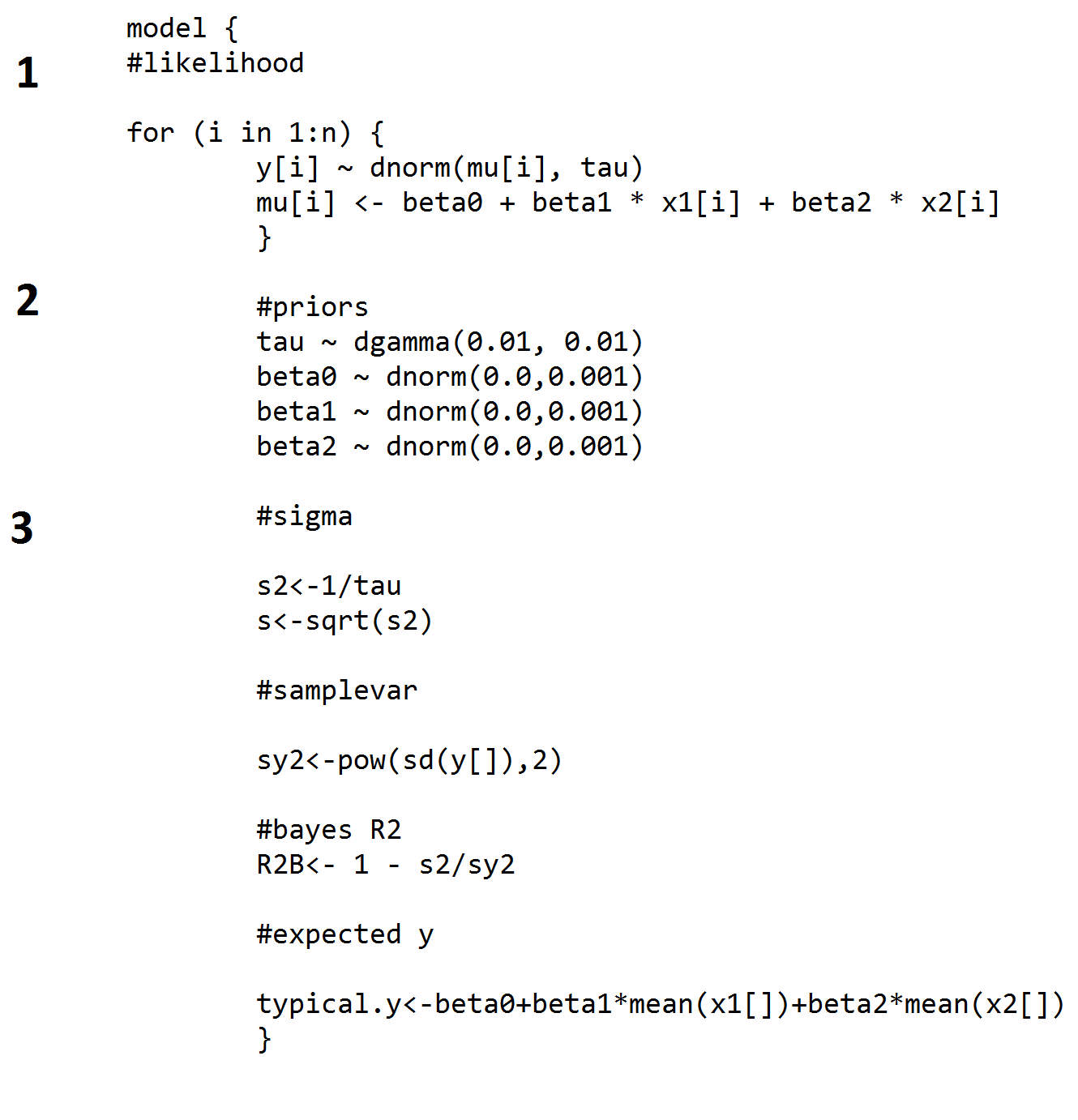# RJAGS: how to get started

## Solutions

Developed by Naomi Schalken and Rens van de Schoot

This tutorial provides the reader with a basic introduction to the software package RJags (Plummer, Stukalov & Denwood, 2016). RJags is a free package that can be used within the R environment. The reader will be guided through the process of downloading Rjags and reading and running the R code to conduct a Bayesian regression analysis. Most of the written text consists of instructions. The exercises that are described in this document can also be found in the RegressionRjags.r file, which includes all the commands to run.

### Exercise 1

Open RStudio and open the .R file (RegressionRjags.r) that’s included in the folder. Then follow the next steps:

c. Split the data in vectors for each variable, create a vector that holds the length of the data (N).
d. Define initial values for the model parameters of interest.
e. Specify number of iterations and number of burn-in iterations (that will not be included for posterior).

### Exercise 2

In this exercise we continue with running the JAGS model. Before we run the model in R, open the Regression2JAGS.txt file in the folder. Take a look at the code in the file (see image below). The code is explained in three steps below.1. First, we specify the model, and start with the likelihood (a for-loop). Y is a normally distributed variable with mu (specified in the code) as the mean and tau as the precision. OpenBugs works with precision instead of variance. Precision is the inverse of the variance.

2. Underneath the likelihood we specify the priors to use for this model. The priors used here are relatively uninformative, with normal distributions for the intercept (beta0) and regression coefficients (beta1 and beta2), and a gamma distribution for the precision.

3. Then we define a few more parameters. First, the residual variance of y (s2), and the square root of this variance, the standard deviation (s). Below that we use a formula to retrieve the total variance of y (sy2) and use that to compute a “Bayesian R2”. Finally, we compute a typical y value based on the regression coefficients, using mean values for the observed x1 and x2.

Task a: Run the JAGS model. The txt. file holds the Bugs model input (excluding data and inits parts). Data is a list of the vectors you specified in Exercise 1. N.chains are the number of chains you specified in Exercise 1.

Task b: Run the model for a certain number of MCMC iterations and extract random samples from the posterior distribution of parameters of interest. First specify the model object and then c() the parameters you would like to have information on. N.iter is the number of iterations to monitor. We specified this number in Exercise 1.

Task c: Obtain summary estimates of the parameters. What can be concluded about these parameters?

Task d: Obtain plots of the posterior distributions of the parameters.

### References

Plummer, M., Stukalov, A., & Denwood, M. (2016). Bayesian Graphical Models using MCMC.